# module ml.voronoi#

## Short summary#

module mlstatpy.ml.voronoi

source on GitHub

## Classes#

class

truncated documentation

VoronoiEstimationError

Raised when the algorithm failed.

## Functions#

function

truncated documentation

voronoi_estimation_from_lr

Determines a Voronoi diagram close to a convex partition defined by a logistic regression in n classes.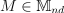## Documentation#

source on GitHub

exception mlstatpy.ml.voronoi.VoronoiEstimationError#

Bases : Exception

Raised when the algorithm failed.

source on GitHub

mlstatpy.ml.voronoi.voronoi_estimation_from_lr(L, B, C=None, D=None, cl=0, qr=True, max_iter=None, verbose=False)#

Determines a Voronoi diagram close to a convex partition defined by a logistic regression in n classes.a row matrix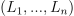. Every border between two classes i and j is defined by: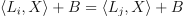.

The function looks for a set of points from which the Voronoi diagram can be inferred. It is done through a linear regression with norm L1. See Régression logistique, diagramme de Voronoï, k-Means.

Paramètres:
• L – matrix

• B – vector

• C – additional conditions (see below)

• D – addition condictions (see below)

• cl – class on which the additional conditions applies

• qr – use quantile regression

• max_iter – number of condition to remove until convergence

• verbose – display information while training

Renvoie:

matrix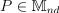The function solves the linear system: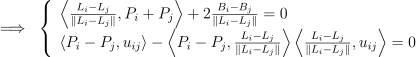If the number of dimension is big and the number of classes small, the system has multiple solution. Addition condition must be added such as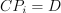where i=cl,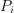is the Voronoï point attached to class cl. Quantile regression is not implemented in scikit-learn. We use QuantileLinearRegression.

After the first iteration, the function determines the furthest pair of points and removes it from the list of equations. If max_iter is None, the system goes until the number of equations is equal to the number of points * 2, otherwise it stops after max_iter removals. This is not the optimal pair to remove as they could still be neighbors but it should be a good heuristic.

source on GitHub